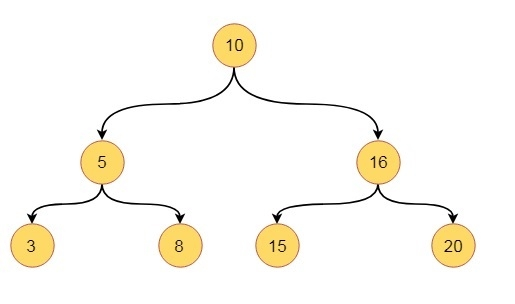# Binary Trees and Properties in Data Structures

In this section we will see some important properties of one binary tree data structure. Suppose we have a binary tree like this.Some properties are −

• The maximum number of nodes at level ‘l’ will be $2^{l-1}$ . Here level is the number of nodes on path from root to the node, including the root itself. We are considering the level of root is 1.
• Maximum number of nodes present in binary tree of height h is $2^{h}-1$ . Here height is the max number of nodes on root to leaf path. Here we are considering height of a tree with one node is 1.
• In a binary tree with n nodes, minimum possible height or minimum number of levels are$\log_{2}\lgroup{n+1}\rgroup$ . If we consider that the height of leaf node is considered as 0, then the formula will be $\log_{2}\lgroup{n+1}\rgroup-1$
• A binary tree with ‘L’ leaves has at least $\log_{2}{L+1}$ number of levels
• If a binary tree has 0 or 2 children, then number of leaf nodes are always one more than nodes with two children.

N.B. As binary tree is one kind of tree; it has all properties of tree in graph theory.